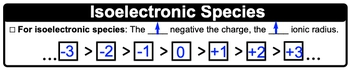Start typing, then use the up and down arrows to select an option from the list.## General Chemistry

Learn the toughest concepts covered in Chemistry with step-by-step video tutorials and practice problems by world-class tutors

10. Periodic Properties of the Elements

Ionic Radius is the distance between an ion's nucleus and its most outer occupied electron shell.

1
concept2m
Play a video:

Periodic Trend: Ionic Radius increases as the number of electrons increases.

2
example

## Periodic Trend: Ionic Radius Example 11m
Play a video:
3
example

## Periodic Trend: Ionic Radius Example 23m
Play a video:
here, we're going to say when ranking Ionic Radio I you must determine the total number of electrons for atoms and ions. Now Mawr electrons equals larger Ionic radius. But recall is Elektronik. Species are atoms or ions that have the same number of electrons in those cases. What do we do? Well, if we take a look at this example, it says, arranged the atoms and her ions in order of decreasing Ionic radius. So that means that we need to go from largest Ionic Radius two smallest Ionic radius. So here, if we take a look in step one, it says determined the total number of electrons for each element or ion. Just remember the higher the number of electrons than the greater the Ionic radius. So if we take a look here iron when it is neutral, it has 26 electrons because its atomic number is 26 here. It's lost to, so it's gonna have 24 electrons remaining. Mango knees has an atomic number of 25 so when it's neutral, it has 25 electrons plus one me that's lost one, so it has 24 electrons as well. Nickel has an atomic number of 28 here. It's just lost two electrons, so it has 26 remain. And then finally, here we have zinc. Zinc has an atomic number of 30 so when it's neutral, it has 30 electrons, plus two means. Now it's lost to, so it only has 28 electrons remaining. All right, so we can arrange this somewhat from largest to smallest. We know that zinc two plus ion will be the largest because it has the most electrons, followed by nickel. But how do we break the tie between iron two plus and manganese plus one? That's where Step two comes into play. If the atoms or ions have equal electrons, meaning there is Elektronik, then the more negative the charge, the larger the Ionic radius. So we're gonna say for an Ionic for is electric species We just said the greater than negative charge than the greater the Ionic Radius. So a negative three charge will be bigger than a negative to charge bigger than a negative one bigger than zero bigger than plus one plus two plus three. Now, of course, you could have some species that have a charge that's outside of negative three or beyond plus three is well, same rules would apply. So both iron two plus and manganese plus one have 24 electrons. But Mangga Nis plus one is bigger than plus two. So we'd say that Mangga Nis plus one ion is bigger than iron two plus ion. So just remember, when it comes to ions mawr electrons equals larger ionic radius. If they're tied, then we look at the charge to break that tie, the more negative the charge, the larger die Ionic radius will be.4
Problem

Arrange the following atoms and/or ions in the order of increasing size:Br –, Kr, Rb+, Sr2+.

5
Problem

For an isoelectronic series of ions, the ion that is the smallest is always